# OASP笔记 - 8

## 连续吸收系数

Posted by mingjie on January 2, 2018

### 中性氢

##### 束缚-自由跃迁

$\lambda$以埃为单位时$\alpha_0 = 1.0449\times10^{-26}$$g_\mathrm{bf}$是从经典转换到量子的因子，接近1。Karzas and Latter (1961)给出了一个表，但是一般来说用Menzel and Pekris (1935)的公式就足够了：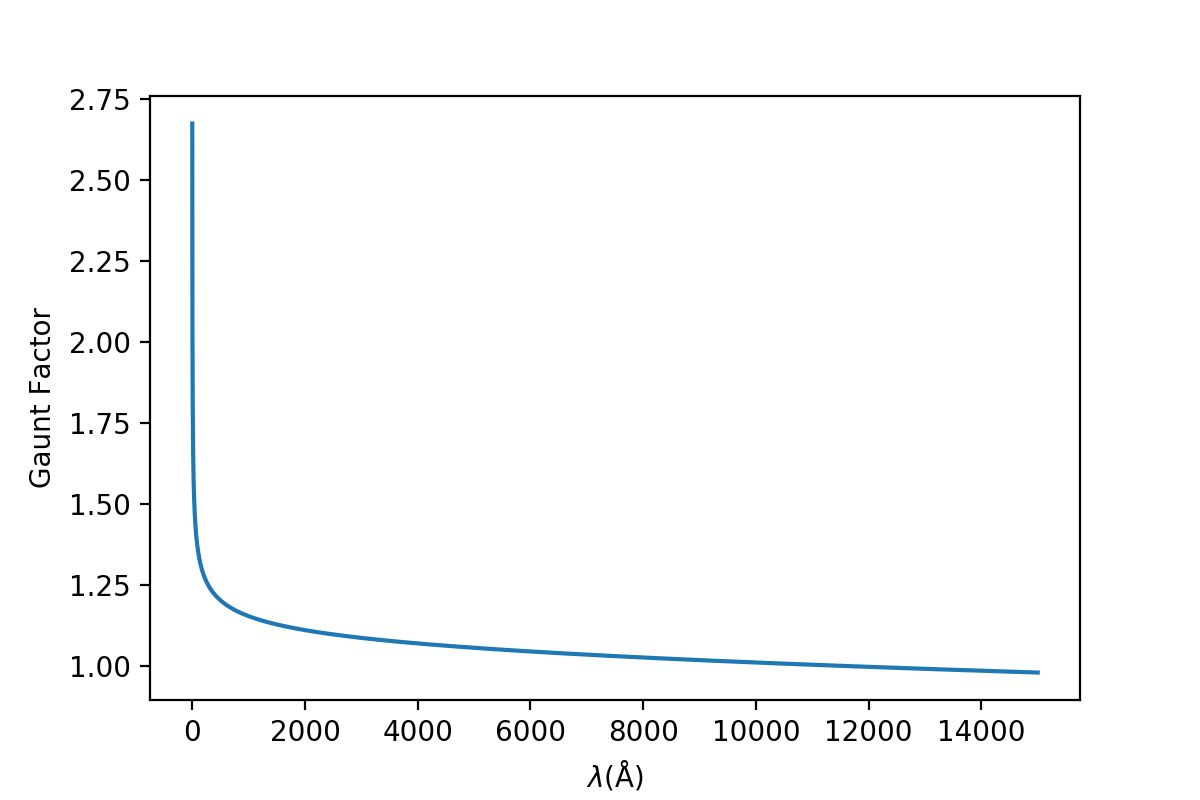束缚-自由过程的Gaunt因子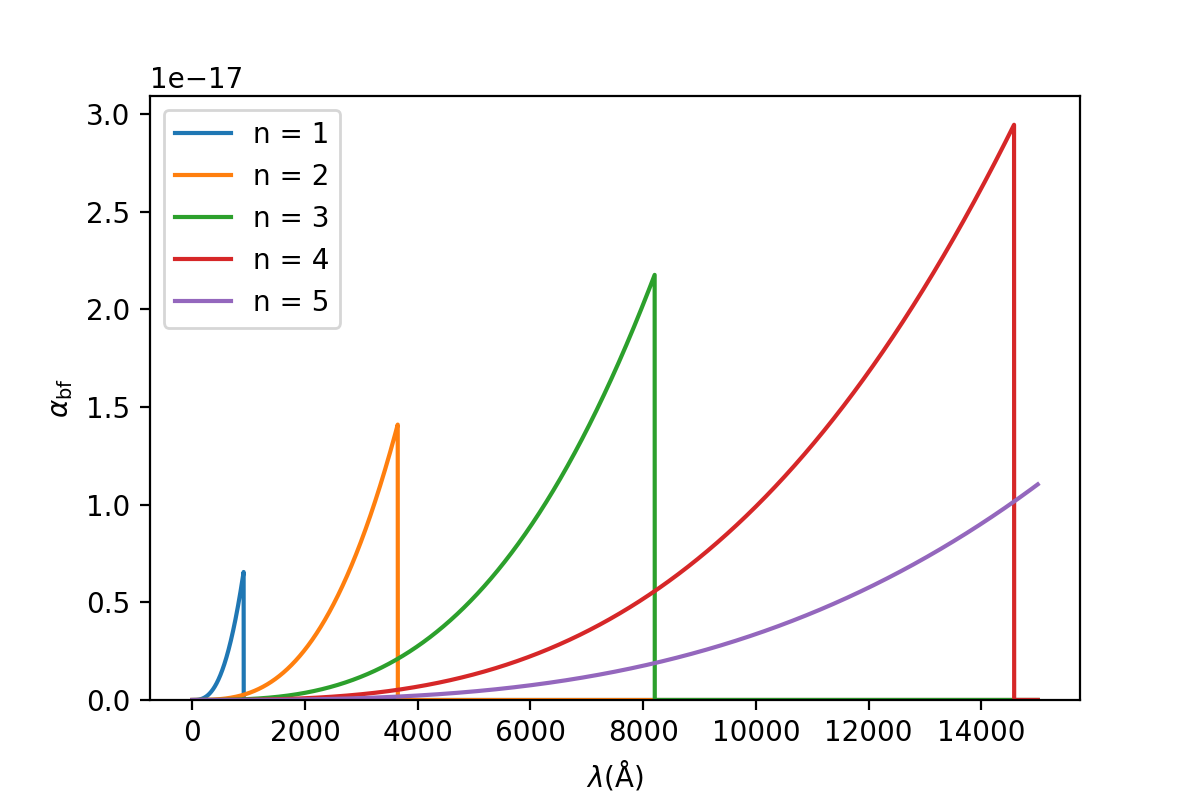(线系限波长取自维基百科)

Unfold (1995)证明了大于$n_0 + 2$的项之和可以用一个积分来代替：

$(8.3)$$d\chi = -Id(1/n^2)$，所以

### 负氢离子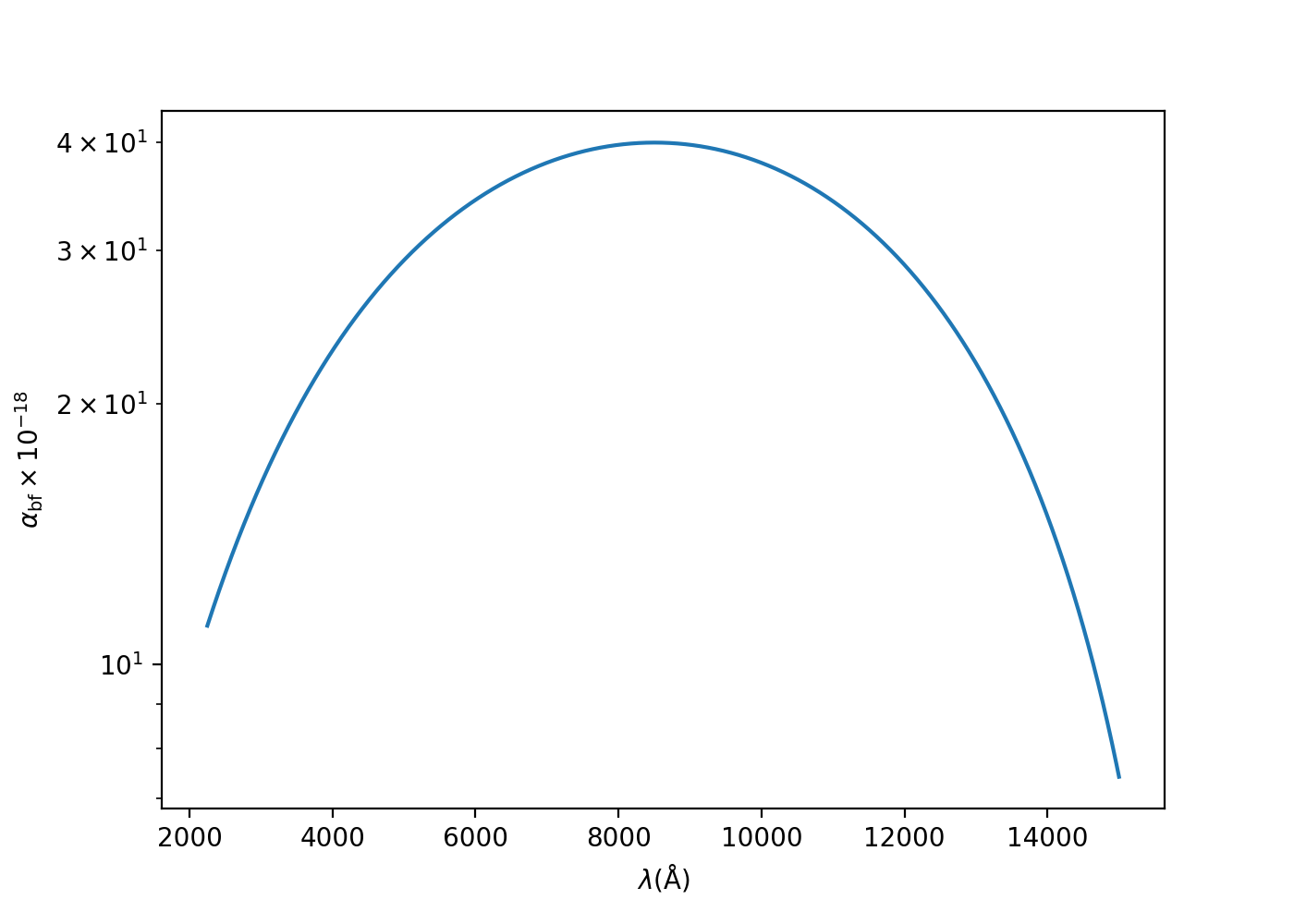### 小结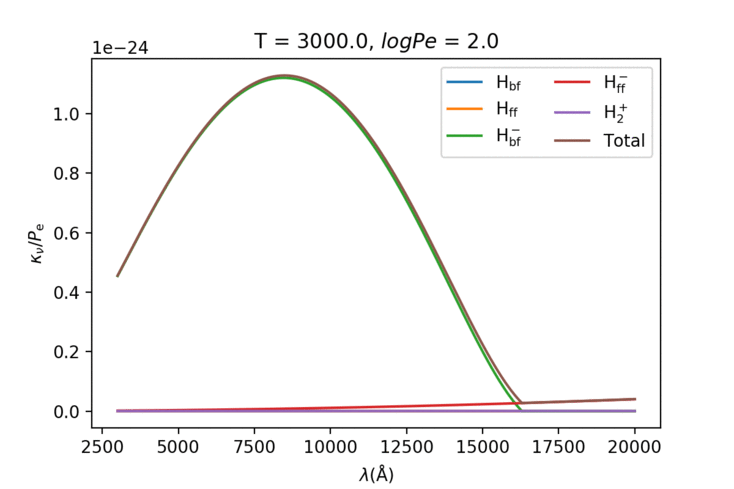• 算氢原子的bf的时候只算6项不够，要算100项左右；
• 配分函数怎么具体算还不是很明确导致$\mathrm{He_{ff}^-}$没加进去（虽然都是可以忽略的）；可能需要估计各种元素丰度？

### 电子的Thomson散射

$A_\mathrm{j} = N_\mathrm{j} - N_\mathrm{H}$，所以

### 其他的吸收源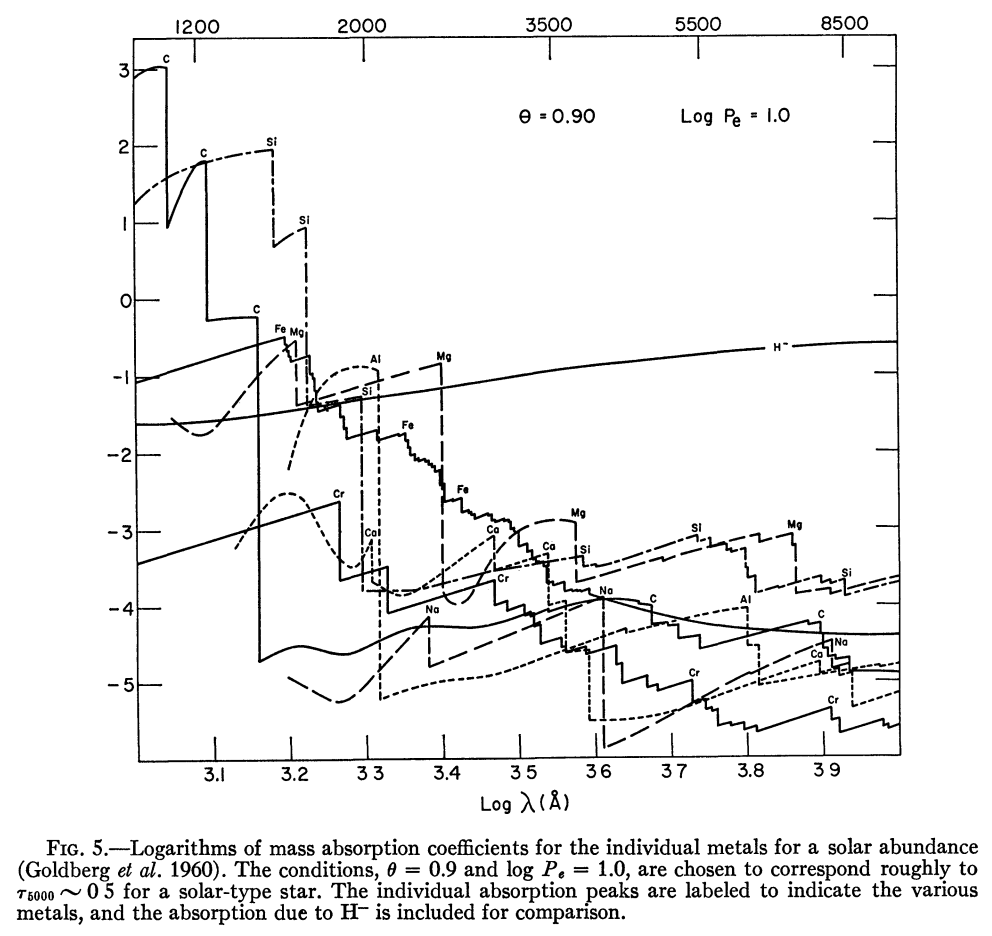### 总连续吸收系数

$\sum A_j\mu_j$的典型值为$2.0\times10^{-24}$g/H。